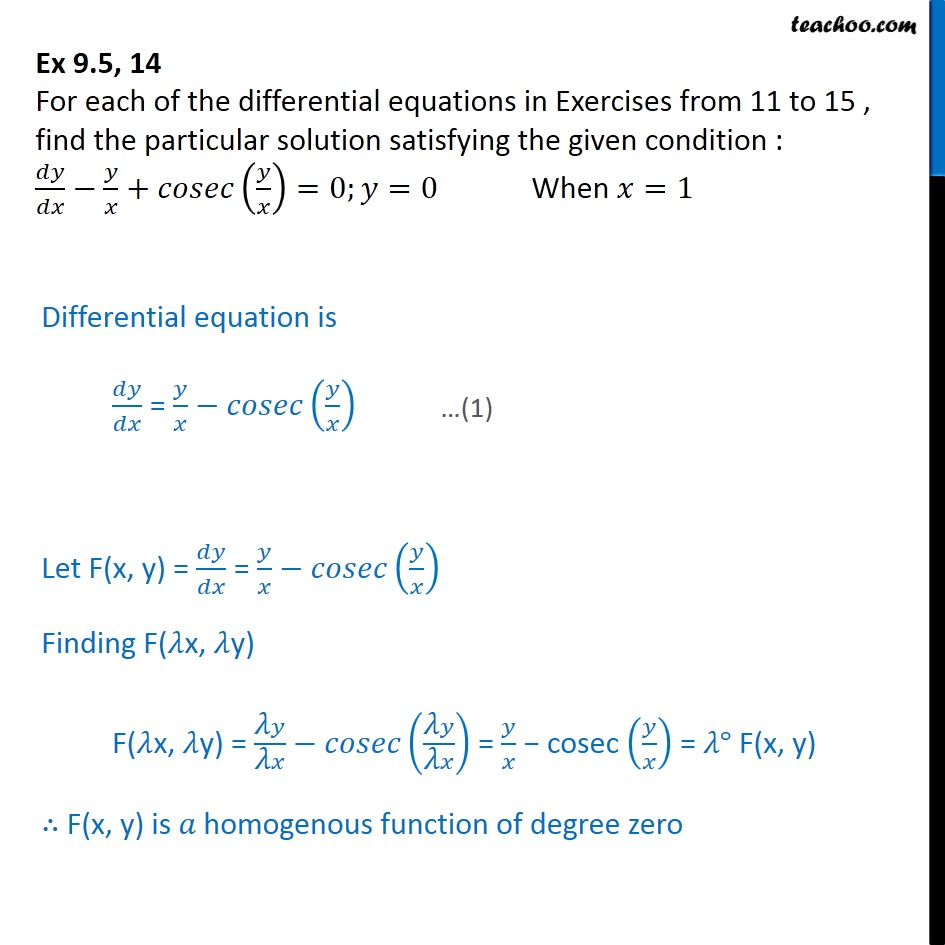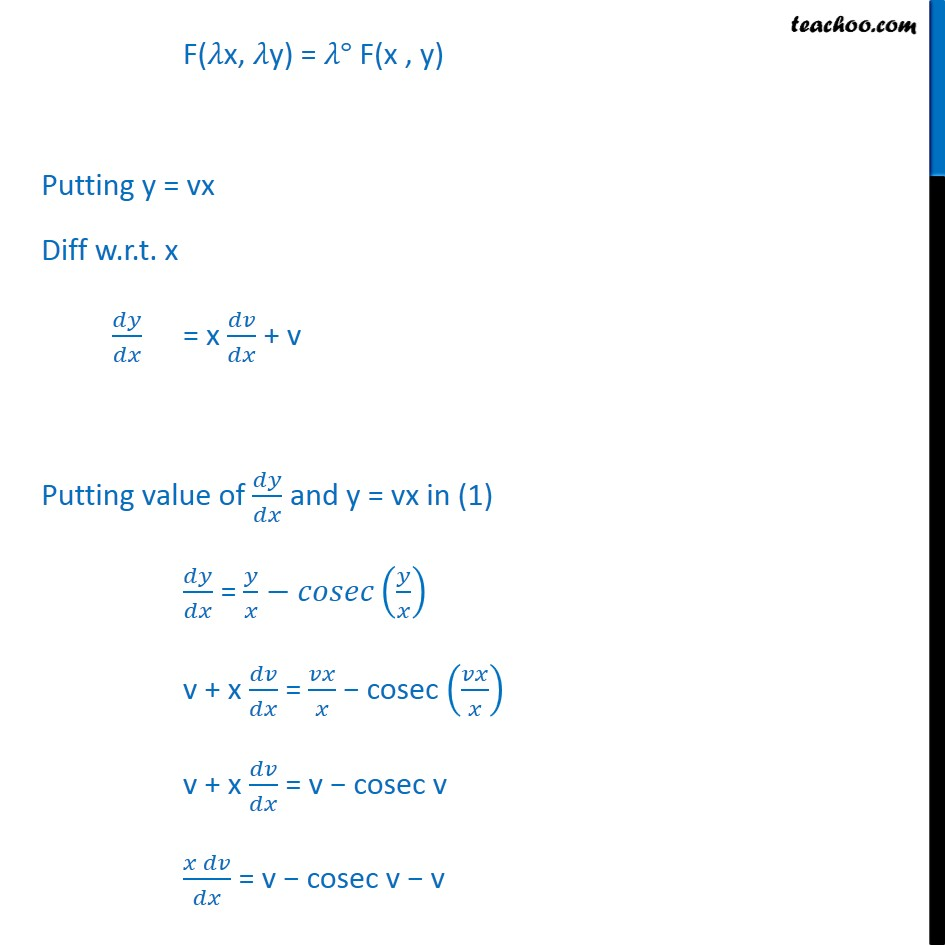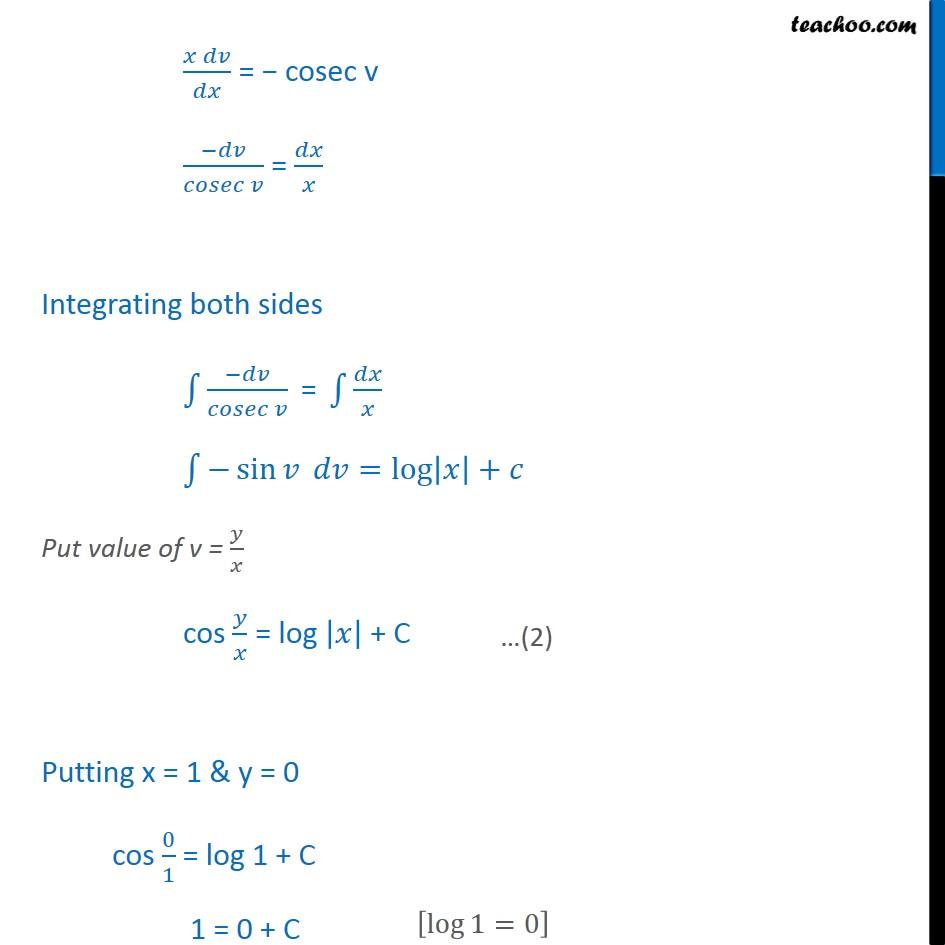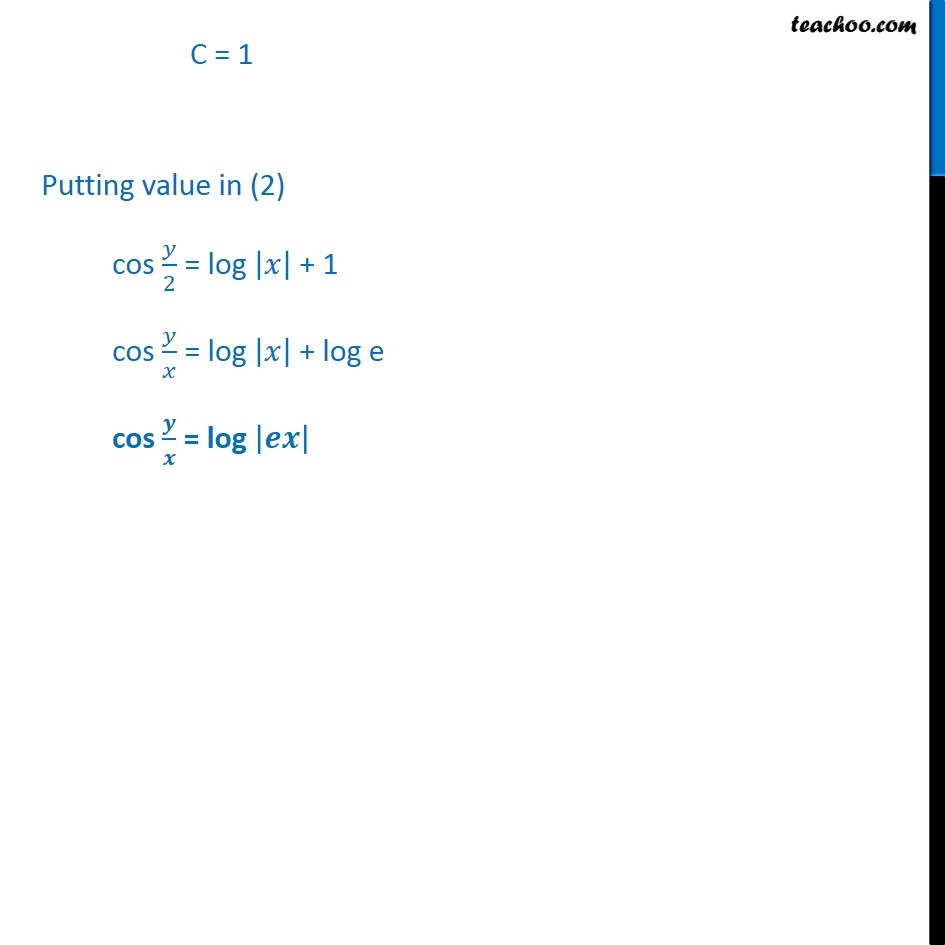Ex 9.4

Chapter 9 Class 12 Differential Equations
Serial order wiseLearn in your speed, with individual attention - Teachoo Maths 1-on-1 Class

### Transcript

Ex 9.4, 14 For each of the differential equations in Exercises from 11 to 15 , find the particular solution satisfying the given condition : 𝑑𝑦/𝑑𝑥−𝑦/𝑥+𝑐𝑜𝑠𝑒𝑐(𝑦/𝑥)=0;𝑦=0 When 𝑥=1 Differential equation is 𝑑𝑦/𝑑𝑥 = 𝑦/𝑥−𝑐𝑜𝑠𝑒𝑐(𝑦/𝑥) Let F(x, y) = 𝑑𝑦/𝑑𝑥 = 𝑦/𝑥−𝑐𝑜𝑠𝑒𝑐(𝑦/𝑥) Finding F(𝜆x, 𝜆y) F(𝜆x, 𝜆y) = ("𝜆" 𝑦)/("𝜆" 𝑥)−𝑐𝑜𝑠𝑒𝑐(("𝜆" 𝑦)/("𝜆" 𝑥)) = 𝑦/𝑥 − cosec (𝑦/𝑥) = 𝜆° F(x, y) ∴ F(x, y) is 𝑎 homogenous function of degree zero F(𝜆x, 𝜆y) = 𝜆° F(x , y) Putting y = vx Diff w.r.t. x 𝑑𝑦/𝑑𝑥 = x 𝑑𝑣/𝑑𝑥 + v Putting value of 𝑑𝑦/𝑑𝑥 and y = vx in (1) 𝑑𝑦/𝑑𝑥 = 𝑦/𝑥−𝑐𝑜𝑠𝑒𝑐(𝑦/𝑥) v + x 𝑑𝑣/𝑑𝑥 = 𝑣𝑥/𝑥 − cosec (𝑣𝑥/𝑥) v + x 𝑑𝑣/𝑑𝑥 = v − cosec v (𝑥 𝑑𝑣)/𝑑𝑥 = v − cosec v − v (𝑥 𝑑𝑣)/𝑑𝑥 = − cosec v (−𝑑𝑣)/(𝑐𝑜𝑠𝑒𝑐 𝑣) = 𝑑𝑥/𝑥 Integrating both sides ∫1▒〖(−𝑑𝑣)/(𝑐𝑜𝑠𝑒𝑐 𝑣) " = " ∫1▒𝑑𝑥/𝑥〗 ∫1▒〖−sin⁡𝑣 𝑑𝑣〗=log⁡〖|𝑥|+𝑐〗 Put value of v = 𝑦/𝑥 cos 𝑦/𝑥 = log |𝑥| + C Putting x = 1 & y = 0 cos 0/1 = log 1 + C 1 = 0 + C C = 1 Putting value in (2) cos 𝑦/2 = log |𝑥| + 1 cos 𝑦/𝑥 = log |𝑥| + log e cos 𝒚/𝒙 = log |𝒆𝒙|